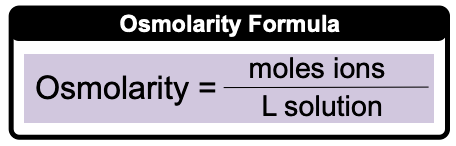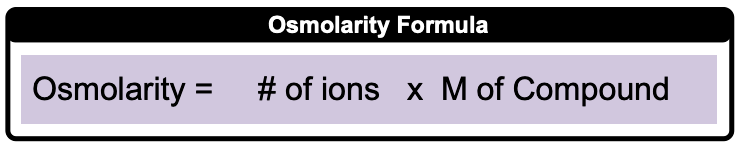Clutch Prep is now a part of Pearson

# Osmolarity

See all sections
Sections
Solutions
Solubility and Intermolecular Forces
Solutions: Mass Percent
Percent Concentrations
Molarity
Osmolarity
Parts per Million (ppm)
Solubility: Temperature Effect
Intro to Henry's Law
Henry's Law Calculations
Dilutions
Solution Stoichiometry
Electrolytes (Simplified)
Equivalents
Molality
The Colligative Properties
Boiling Point Elevation
Freezing Point Depression
Osmosis
Osmotic Pressure

Osmolarity (ionic molarity) represents the number of moles of ions per liter of solution.

###### Osmolarity

Concept #1: Method 1: Direct Calculation of Osmolarity

Direct Calculation of Osmolarity:Example #1: Calculate the molarity of chloride ions when dissolving 58.1 g AlCl3 in enough water to make 500 mL of solution.

Concept #2: Method 2: Osmolarity from Molarity

Osmolarity from Molarity of compound:Example #2: What is the concentration of hydroxide ions in a 0.350 M solution of gallium hydroxide, Ga(OH)3?

Example #3: How many moles of Ca2+ ions are in 0.120 L of 0.450 M Ca3(PO4)2 solution?

Using Molarity of compounds and L of solutions, moles of ions can be calculated.

Practice: Which of the following solutions will have the highest concentration of bromide ions?

Practice: How many milligrams of nitride ions are required to prepare 820 mL of 0.330 M Ba3N2 solution?

Practice: How many bromide ions are present in 65.5 mL of 0.210 M GaBrsolution?